# C Program to Print Odd Numbers in an Array

In this tutorial, we will learn how to create a program in C that will ask the user to enter array elements and then print out all the odd numbers or elements from the given array. Here is the program:

```#include<stdio.h>
#include<conio.h>
int main()
{
int arr, i;
printf("Enter any 10 array elements: ");
for(i=0; i<10; i++)
scanf("%d", &arr[i]);
printf("\nOdd Array elements are:\n");
for(i=0; i<10; i++)
{
if(arr[i]%2!=0)
{
printf("%d ", arr[i]);
}
}
getch();
return 0;
}```

As the above program was written in the Code::Blocks IDE, here is the first snapshot of the sample run: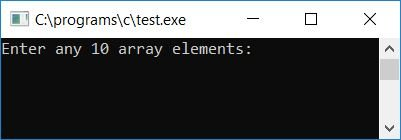Provide any 10 array elements and then press the ENTER key to see the output that will list out all the odd elements from the list of given 10 array elements, as shown here in the second snapshot: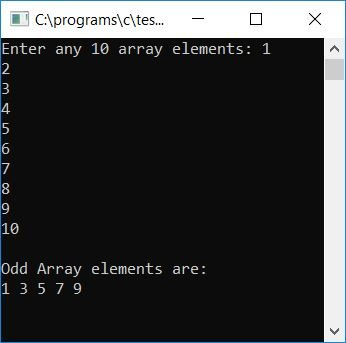#### Program Explained

• Receive any 10 numbers as 10 array elements.
• Create a for loop that starts from 0 to 9, as indexing starts from 0.
• Inside the for loop, check whether the element or number present at the current array index is an odd number or not.
• If it is an odd number, then print it out; otherwise, continue to check for the next odd array element.
• In this way, all the odd numbers get printed on the output screen.

### Copy odd numbers to another array

Now let's modify the above program and create another program that will create another array containing all odd array elements of the original array (provided by the user):

```#include<stdio.h>
#include<conio.h>
int main()
{
int arr, i, b, j=0, count=0;
printf("Enter any 10 array elements: ");
for(i=0; i<10; i++)
scanf("%d", &arr[i]);
for(i=0; i<10; i++)
{
if(arr[i]%2!=0)
{
b[j] = arr[i];
count++;
j++;
}
}
printf("\nOdd elements are:\n");
for(i=0; i<count; i++)
{
if(i==(count-1))
printf("%d", b[i]);
else
printf("%d, ", b[i]);
}
getch();
return 0;
}```

After a successful build and run, here is the first snapshot of the sample run: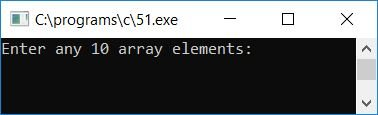Provide any 10 array elements and then press the ENTER key to see the following output: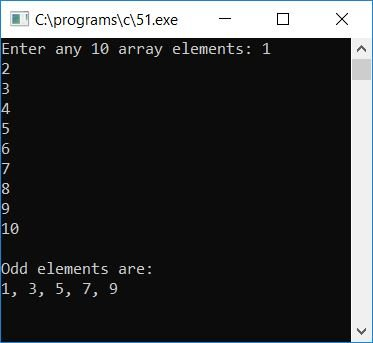#### Program Explained

• Receive any 10 array elements.
• Create a for loop that starts from 0 to 9.
• Check whether the current element is an odd number or not.
• If it is an odd number, then place this array element inside another array, say b[].
• Indexing starts in array b[] (where all the odd numbers of the original array will get placed) and starts with 0.
• Therefore, the variable provided here, say j, is initialized with 0 and then incremented by one each time the odd number from the original array gets placed inside the second array, say b[].
• In addition, increment the value of a variable, say count (which is responsible for the length of the second array, say b[]).
• After checking each element of the original array and inserting the element with an odd number into the second array, come out of this loop.
• Make another for the loop and print all the values of the second array.
• Here the array b[] contains all the odd array elements present inside the original array provided by the user.
• In this way, we will see all the odd array elements on the output screen.

### Allow the user to change the size of the array

Let's modify the above program to allow the user to define the array size along with its elements:

```#include<stdio.h>
#include<conio.h>
int main()
{
int arr, i, b, j=0, count=0, size;
printf("Enter array size: ");
scanf("%d", &size);
printf("Enter any %d array elements: ", size);
for(i=0; i<size; i++)
scanf("%d", &arr[i]);
for(i=0; i<size; i++)
{
if(arr[i]%2!=0)
{
b[j] = arr[i];
count++;
j++;
}
}
printf("\nOdd elements are:\n");
for(i=0; i<count; i++)
{
if(i==(count-1))
printf("%d", b[i]);
else
printf("%d, ", b[i]);
}
getch();
return 0;
}```

Here is the final snapshot of the sample run: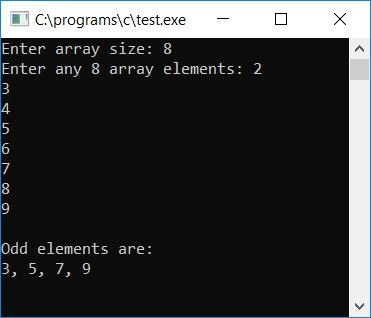The logic in the preceding program is nearly identical, with the exception that we have used the "size" variable (which holds the size for the array provided by the user at run-time) instead of 10, implying that the user is allowed to enter the size for the array and then elements for the same array.

C Quiz

« Previous Program Next Program »